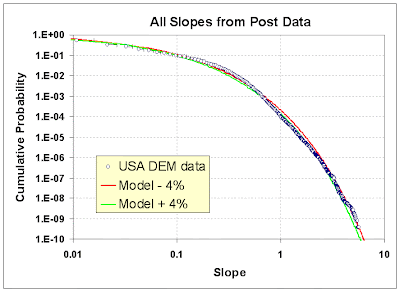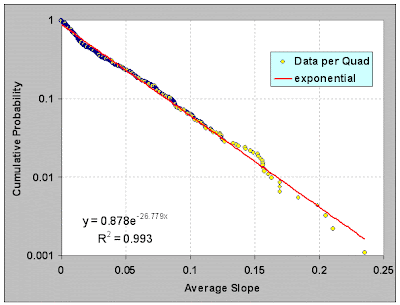[[ Check out my Wordpress blog Context/Earth for environmental and energy topics tied together in a semantic web framework ]]

## Saturday, January 08, 2011

### Terrain Slopes

Entropy makes its mark everywhere. Take the case of modeling topography. How can we model and thus characterize disorder in the earth's terrain? Can we actually understand the extreme variability we see?

If we consider that immense forces cause upheaval in the crust then we can reason that the energy can also vary all over the map, so to speak. The process that transfers potential energy into kinetic energy to first order has to contain elements of randomness. To the huge internal forces within the earth, generating relief textures equates to a kind of brownian motion in relative terms -- over geological time, the terrain amounts to nothing more than inconsequential particles to the earth's powerful internal engine.

In a related sense the process also resembles the pressure distribution in the earth's atmosphere, a classic application of maximum entropy that we can re-apply in the case of modeling terrain slope distributions.

Premise. We take the terrain slope S as our random variable (defined as rise/run). The higherthe slope, the more energetic the terrain. Applying Maximum Entropy to a section of terrain, we can approximate the local variations as a MaxEnt conditional probability density function:
p(S|E) = (1/cE) * exp(-S/cE)
where E is the local mean energy and c is a constant of proportionality. But we also assume that the mean E varies over a larger area that we are interested in, as in the superstatistical sense of applying a prior distribution.
p(E) = k*exp(-k*E)
where k is another MaxEnt measure of our uncertainty in the energy spread over a larger area.

The final probability is an integral over the marginal distribution consisting of the conditional multiplied by the prior:
p(S) = integral p(S|E) *p(E) dE from E=0 to infinity
This integrates as a BesselK function of the zero order, K0, available on any spreadsheet program (see here for a similar derivation in an unrelated field).
p(S) = 2/S0 * K0(2*sqrt(S/S0))
The average value of the terrain slope for this distribution is simply the value S0.

Now we can try it on a large set of data. I downloaded all the DEM data for the 1 degree quadrangles (aka blocks/tiles) in the USA from the USGS web site. http://dds.cr.usgs.gov/pub/data/DEM/250/

This consists of post data at approximately 92 meter intervals (i.e. a fixed value of run) at 1:250,000 scale for the entire USA. I concentrated on the lower 48 and some spillover into Canada. I used curl to iteratively download each of the nearly 1000 quadrangle files on the server.

I then wrote a program to read the data from individual DEM files and calculate the slopes between adjacent posts and came up with an average slope (rise/run) of 0.039, approximately a 4% grade or 2.2 degrees pitch. I take the absolute values of all slopes so that the average is not zero.

The cumulative plot of terrain slopes for all 5 billion calculated slope points appears on the following chart (Figure 1). I also added the cumulative probability distribution of the BesselK model with the calculated average slope as the single adjustable parameter.Figure 1: CDF of USA DEM data and the BesselK model with a small variation in S0 (+/-4% about the average 0.037 rise/run) demonstrating sensitivity to the fit.

This kind of agreement does not just happen because of coincidence. It occurs because random forces contribute to maximizing the entropy of the topography. Enough variability exists for the terrain to reach an ergodic limit in filling the energy-constrained state space.

As supporting evidence, it turns out that we can generate a distribution that maps well to the prior by estimating the average slope from the conditional PDF of each of the 922 quadrangle blocks and then plotting this aggregate data set as another histogram (see Figure 2).Figure 2: Generation of the prior distribution by taking the average slope of each of the nearly 1000 quadrangles . The best fit generates a value of S0 (1/27=0.037) close to that used in Figure 1.

Practically speaking, we see the variability in slopes expressed at the two different levels. The entire USA at the integrated (BesselK model) level and the aggregated regions at the localized (exponential prior) level. These remain consistent as they agree on the single adjustable parameter S0 .

The modeled distribution has many practical uses for analysis, including transportation studies and planning. Obviously, vehicles traveling up slopes use a significant amount of energy and you might like to have a model to base an analysis on without having to rely on the data by itself. (As a caveat, I did not include any of the spatial correlations that must also exist and might prove useful as well)

Perusing the recent research, I couldn't find anyone that had previously discovered this simple model. Not that they haven't tried, coming up with a good slope distribution model seems to amount to a mini Holy Grail among geophysicists. I went as far as dropping \$10 to downloading the first paper, which turned out to be a bust.
1. Probabilistic description of topographic slope and aspect.
G. Vico and A. Porporato, JOURNAL OF GEOPHYSICAL RESEARCH, VOL. 114, F01011, doi:10.1029/2008JF001038, 2009
http://www.agu.org/journals/jf/jf0901/2008JF001038/2008JF001038.pdf
2. Nonlinear Processes in Geophysics Multifractal earth topography.
J.-S. Gagnon, S. Lovejoy, and D. Schertzer, Nonlin. Processes Geophys., 13, 541–570, 2006
http://hal.archives-ouvertes.fr/docs/00/33/10/93/PDF/npg-13-541-2006.pdf
3. PROPAGATION OF DEM UNCERTAINTY: AN INTERVAL ARITHMETIC APPROACH.
G Gonçalves, XXII International Cartographic Conference, 2005
http://www.cartesia.org/geodoc/icc2005/pdf/oral/TEMA7/Session%202/GIL%20GON%C7ALVES.pdf
4. SAR interferometry and statistical topography.
Guarnieri, A.M. IEEE Transactions on Geoscience and Remote Sensing, Dec 2002
http://home.dei.polimi.it/monti/papers/montiguarnieri02.pdf
If someone wants to generate Monte Carlo statistics for the BesselK model without having to do the probability inversion, the algorithm turns out surprisingly simple. Draw two independent random samples from a uniform [0.0 .. 1.0] interval, apply the natural log to each, multiply them together, and then multiply by the S0 scaling constant. That will give the following cumulative if done 5 billion times, which is the same size as my USA DEM data sample.

The only statistical noise is at the 1e-9 level, same as in the DEM data.

Examples of some random-walk realizations drawing from a two-level model follow. The flatter regions occur more often reflecting the regional data.